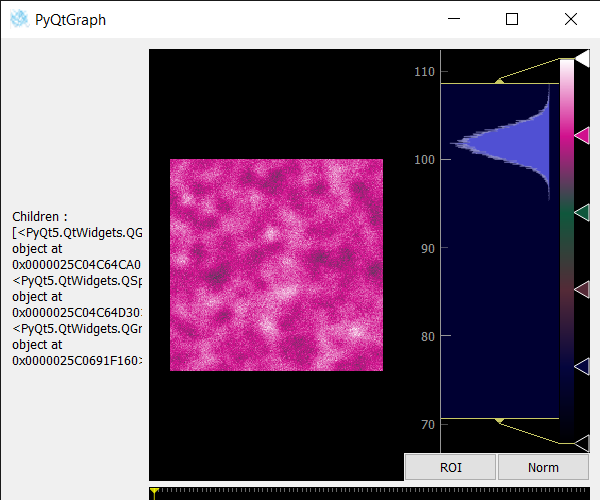Open in App
Not now

# PyQtGraph – Getting Children of Image View

• Last Updated : 25 May, 2022

In this article, we will see how we can get the children of the image view object in PyQTGraph. The PyQtGraph is a graphics and user interface library for Python that provides functionality commonly required in designing and science applications. Its primary goals are to provide fast, interactive graphics for displaying data (plots, video, etc.), widgets used for display and analysis of image data, implements many features like displaying 2D and 3D image data. For 3D data, a z-axis slider is displayed allowing the user to select which frame is displayed. Displays histogram of image data with a movable region defining the dark/light levels, editable gradient provides a color lookup table. Image view is a group of widgets like it contains a view object, push-button and scroll bars, etc.

We can create an image view object with the help of command given below

```# creating a pyqtgraph image view object
imv = pg.ImageView()```

In order to get children of Image View object, we use children() method with the image view object.

Syntax : imv.children()
Argument : It takes no argument
Return : It returns list

Below is the program to get children of an Image view using PyQTGraph module:

## Python3

 `# importing Qt widgets` `from` `PyQt5.QtWidgets ``import` `*`   `# importing system` `import` `sys`   `# importing numpy as np` `import` `numpy as np`   `# importing pyqtgraph as pg` `import` `pyqtgraph as pg` `from` `PyQt5.QtGui ``import` `*` `from` `PyQt5.QtCore ``import` `*`     `# Image View class` `class` `ImageView(pg.ImageView):`   `    ``# constructor which inherit original` `    ``# ImageView` `    ``def` `__init__(``self``, ``*``args, ``*``*``kwargs):` `        ``pg.ImageView.__init__(``self``, ``*``args, ``*``*``kwargs)`     `class` `Window(QMainWindow):`   `    ``def` `__init__(``self``):` `        ``super``().__init__()`   `        ``# setting title` `        ``self``.setWindowTitle(``"PyQtGraph"``)`   `        ``# setting geometry` `        ``self``.setGeometry(``100``, ``100``, ``600``, ``500``)`   `        ``# icon` `        ``icon ``=` `QIcon(``"skin.png"``)`   `        ``# setting icon to the window` `        ``self``.setWindowIcon(icon)`   `        ``# calling method` `        ``self``.UiComponents()`   `        ``# showing all the widgets` `        ``self``.show()`   `        ``# setting fixed size of window` `        ``#self.setFixedSize(QSize(600, 500))`   `    ``# method for components` `    ``def` `UiComponents(``self``):`   `        ``# creating a widget object` `        ``widget ``=` `QWidget()`   `        ``# creating a label` `        ``label ``=` `QLabel(``"Geeksforgeeks Image View"``)`   `        ``# setting minimum width` `        ``label.setMinimumWidth(``130``)`   `        ``# making label do word wrap` `        ``label.setWordWrap(``True``)`   `        ``# setting configuration options` `        ``pg.setConfigOptions(antialias``=``True``)`   `        ``# creating image view object` `        ``imv ``=` `ImageView()`   `        ``# Create random 3D data set with noisy signals` `        ``img ``=` `pg.gaussianFilter(np.random.normal(` `            ``size``=``(``200``, ``200``)), (``5``, ``5``)) ``*` `20` `+` `100`   `        ``# setting new axis to image` `        ``img ``=` `img[np.newaxis, :, :]`   `        ``# decay data` `        ``decay ``=` `np.exp(``-``np.linspace(``0``, ``0.3``, ``100``))[:, np.newaxis, np.newaxis]`   `        ``# random data` `        ``data ``=` `np.random.normal(size``=``(``100``, ``200``, ``200``))` `        ``data ``+``=` `img ``*` `decay` `        ``data ``+``=` `2`   `        ``# adding time-varying signal` `        ``sig ``=` `np.zeros(data.shape[``0``])` `        ``sig[``30``:] ``+``=` `np.exp(``-``np.linspace(``1``, ``10``, ``70``))` `        ``sig[``40``:] ``+``=` `np.exp(``-``np.linspace(``1``, ``10``, ``60``))` `        ``sig[``70``:] ``+``=` `np.exp(``-``np.linspace(``1``, ``10``, ``30``))`   `        ``sig ``=` `sig[:, np.newaxis, np.newaxis] ``*` `3` `        ``data[:, ``50``:``60``, ``30``:``40``] ``+``=` `sig`   `        ``# setting image to image view` `        ``# Displaying the data and assign each frame a time value from 1.0 to 3.0` `        ``imv.setImage(data, xvals``=``np.linspace(``1.``, ``3.``, data.shape[``0``]))`   `        ``# Set a custom color map` `        ``colors ``=` `[` `            ``(``0``, ``0``, ``0``),` `            ``(``4``, ``5``, ``61``),` `            ``(``84``, ``42``, ``55``),` `            ``(``15``, ``87``, ``60``),` `            ``(``208``, ``17``, ``141``),` `            ``(``255``, ``255``, ``255``)` `        ``]`   `        ``# color map` `        ``cmap ``=` `pg.ColorMap(pos``=``np.linspace(``0.0``, ``1.0``, ``6``), color``=``colors)`   `        ``# setting color map to the image view` `        ``imv.setColorMap(cmap)`   `        ``# Creating a grid layout` `        ``layout ``=` `QGridLayout()`   `        ``# minimum width value of the label` `        ``label.setFixedWidth(``130``)`   `        ``# setting this layout to the widget` `        ``widget.setLayout(layout)`   `        ``# adding label in the layout` `        ``layout.addWidget(label, ``1``, ``0``)`   `        ``# plot window goes on right side, spanning 3 rows` `        ``layout.addWidget(imv, ``0``, ``1``, ``3``, ``1``)`   `        ``# setting this widget as central widget of the main window` `        ``self``.setCentralWidget(widget)`   `        ``# getting children of image view` `        ``value ``=` `imv.children()`   `        ``# setting text to the label` `        ``label.setText(``"Children : "` `+` `str``(value))`     `# create pyqt5 app` `App ``=` `QApplication(sys.argv)`   `# create the instance of our Window` `window ``=` `Window()`   `# start the app` `sys.exit(App.``exec``())`

Output :My Personal Notes arrow_drop_up
Related Articles